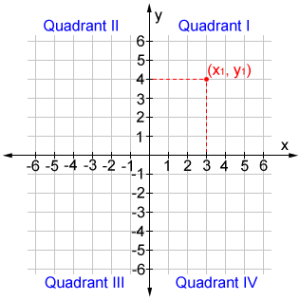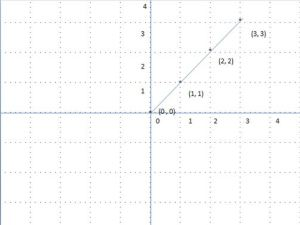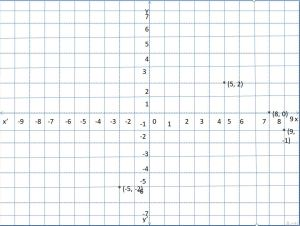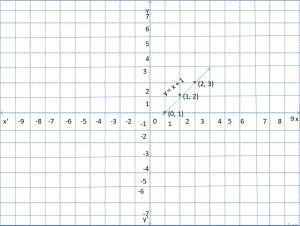# Fundamentals of Coordinate Geometry | Concepts, Coordinate Graph, Quadrants

A plane is any flat surface that can go on infinitely in both directions. Coordinate geometry or analytical geometry is the link between algebra and geometry through graphs having curves and lines. It provides geometric aspects in algebra and enables them to solve geometric problems. Get the detailed information about the coordinate graph, all four quadrants, coordinates of points, others in the following sections.

## Coordinate Geometry Definition

Coordinate geometry is one of the branches of geometry where the position of a point is defined using coordinates. Using the coordinate geometry, you can calculate the distance between two points, find coordinates of a point, plot ordered pairs, and others. The basic terms of coordinate geometry for class 8 students are listed below.

• Coordinate Geometry Definition
• Coordinates of a Coordinate Geometry
• Coordinate Plane

### What is meant by Coordinate and Coordinate Plane?

A coordinate plane is a two-dimensional plane created by the intersection of two axes names horizontal axis (x-axis) and the vertical axis (y-axis). These lines are perpendicular to each other and meet at the point called origin or zero. the axes divide the coordinate plane into four equal sections, and each section is known as the quadrant. The number line which is having quadrants is also known as the cartesian plane.

A set of values that represents the exact position on the coordinate plane is called coordinates. Usually, it is a pair of numbers on the graph denoted as (x, y). Here, x is called the x coordinate, y is called the y coordinate.• Quadrant 1: In this quadrant both x and y are positive. The point is represented as (+x, +y).
• Quadrant 2: X-axis is negative and the y-axis is positive. So, the point is shown as (-x, +y).
• Quadrant 3: Here, both x and y axes are negative. The point in Q3 is represented as (-x, -y).
• Quadrant 4: In this quadrant, x is positive, and y is negative. Coordinates are (+x, -y).

### How to Plot Coordinates of a Point on Graph?

Following are the simple steps to plot coordinates of a point on a graph. Have a look at them and check out how to plot a graph, represent ordered points, and identify signs of axes of a point.

• First of all, take a point which is having both x coordinate ad y coordinate.
• And know the signs of each value in the given point.
• Using those signs, identify under which quadrant the point falls.
• From the respective axes, take those numbers and put a dot on the graph.

### Linear Equation

The general form of a line in the coordinate geometry is Ax + By + C = 0

Where A is the coefficient of x

B is the coefficient of y

And C is the constant value.

Intercept form of a line is y = mx + c. Where (x, y) is a point on the line and m is the slope.

### Graph of Area vs. Side of a Square

To plot a graph of the square area and square side, you need to have the square area for each side length. Measure the square side length each time, find the area by performing the square of side length. Note down those values and take side length on the x-axis, area on the y-axis. As the square side is always positive, the points will automatically come in the first quadrant. Mark points such as side length as x-coordinate and area as y-coordinate for each point in the graph. Join those points to make a graph of area vs square side length.

### Graph of Distance vs. Time

Drawing the graph for area vs side of a square and distance vs time is the same. Here, we are checking the distance traveled by an object in a certain amount of time, what happens when a change happens in either time or distance. Make sure that, the unit of both time and distance must be the same, if not convert them into the same unit. Take distance on the y-axis and time on the x-axis, so the x coordinate will the time, and the y coordinate will be the distance. Mark those points in the first quadrant and join them to draw a graph of distance vs time.

### Example Questions

Example 1.

Plot that the points A (0, 0), B (1, 1), C (2, 2), D (3, 3) and show that these points form a line?

Solution:

Given Points are A (0, 0), B (1, 1), C (2, 2), D (3, 3)

Point A (0, 0) is the origin.From the graph, we can say that the points form a straight line and that line passes through the origin.

Example 2.

Plot each of the following points on a graph?

a. (5, 2) b. (8, 0) c. (-5, -2) d. (9, -1)

Solution:

Given points are (5, 2), (8, 0), (-5, -2), (9, -1)

Coordinates of the point (5, 2) both abscissa and ordinate are positive so the point lies in the first quadrant. On the x-axis, take 5 units to the right of the y-axis and then on the y-axis, take 2 units above the x-axis. Therefore, we get the point (5, 2).

Coordinates of the point (8, 0) both abscissa and ordinate are positive so the point lies in the first quadrant. On the x-axis take 8 units and take 0 units on the y-axis to get the point (8, 0).

For the point (-5, -2), both abscissa and ordinate are negative so the point lies in the third quadrant. Take -5 units on the x-axis and take -2 units on the y-axis to obtain the point (-5, -2).

The point (9, -1) lies in the fourth quadrant because x-coordinate is positive and y-coordinate is negative. To plot this point, take 9 units on the x-axis and take -1 units on the y-axis.Example 3.

Draw the graph of the linear equation y = x + 1?

Solution:

Given linear equation is y = x + 1

The given equation is in the form of y = mx + c

slope m = 1, and constant c = 1

By using the trial and error method, find the value of y for each value of x.

If x = 0, y = 0 + 1, then y = 1

If x = 1, then y = 1 + 1 = 2

If x = 2, then y = 2 + 1 = 3

x 0 1 2
y 1 2 3

Plot the graph for the points mentioned in the above table.

Mark the points (0, 1), (1, 2), (2, 3) on the graph.

Join those points to get a line equation.### FAQs on Coordinate Geometry

1. Why do we need coordinate geometry?

Coordinate geometry has various applications in real life. Some places where we use coordinate geometry is in integration, in digital devices such as mobiles, computes, in aviation to determine the position and location of airplane accurately in GPS, and to map the geographical locations using longitudes and latitudes.

2. Who is the father of Coordinate Geometry?

The father of coordinate geometry is Rene Descartes.

3. What is the name of horizontal and vertical lines that are drawn to find out the position of any point in the Cartesian plane?

The name of horizontal and vertical lines that are drawn to find out the position of any point in the Cartesian plane is determined by the x-axis and y-axis respectively.

4. What is Abscissa and Ordinates in Coordinate Geometry?

Abscissa and Ordinates are used to identify the position of a point on the graph. The horizontal value or x-axis is called the abscissa and the vertical line or the y-axis is called the ordinate. For example, in an ordered pair (1, 8), 2 is abscissa and 8 is ordinate.